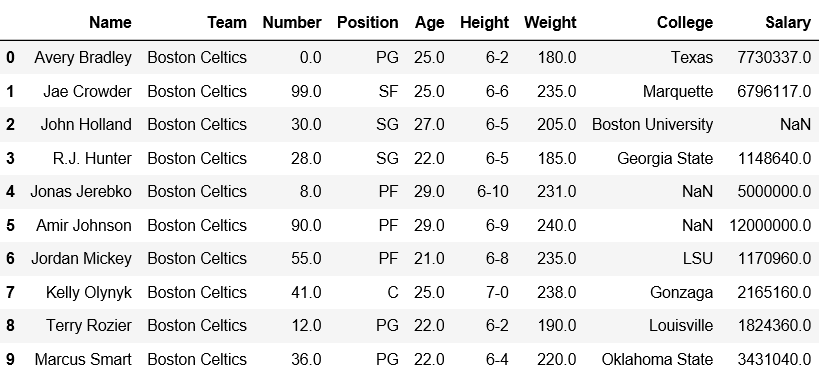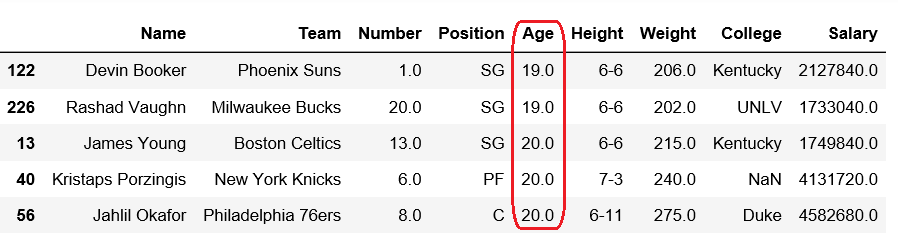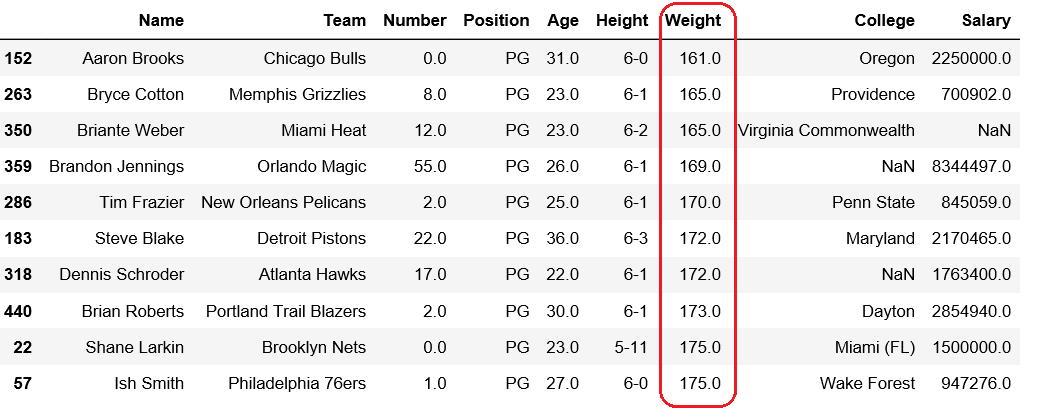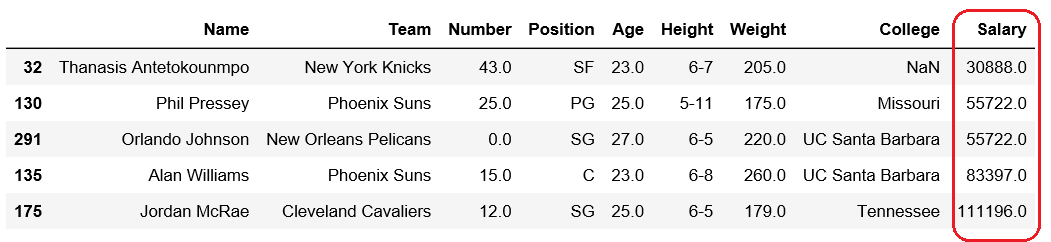Related Articles

# Get n-smallest values from a particular column in Pandas DataFrame

• Last Updated : 18 Dec, 2018

Pandas DataFrame is two-dimensional size-mutable, potentially heterogeneous tabular data structure with labeled axes (rows and columns).

Let’s see how can we can get n-smallest values from a particular column in Pandas DataFrame.

Observe this dataset first. We’ll use ‘Age’, ‘Weight’ and ‘Salary’ columns of this data in order to get n-smallest values from a particular column in Pandas DataFrame.

 `# importing pandas module ``import` `pandas as pd ``   ` `# making data frame ``df ``=` `pd.read_csv(``"https://media.geeksforgeeks.org/wp-content/uploads/nba.csv"``) `` ` `df.head(``10``)`Code #1: Getting 5 smallest Age

 `# importing pandas module ``import` `pandas as pd ``   ` `# making data frame ``df ``=` `pd.read_csv(``"nba.csv"``) `` ` ` ` `df.nsmallest(``5``, [``'Age'``])`

Output:Code #2: Getting 10 minimum weights

 `# importing pandas module ``import` `pandas as pd ``   ` `# making data frame ``df ``=` `pd.read_csv(``"nba.csv"``) `` ` ` ` `df.nsmallest(``10``, [``'Weight'``])`

Output:Code #3: Getting 10 minimum salary

 `# importing pandas module ``import` `pandas as pd ``   ` `# making data frame ``df ``=` `pd.read_csv(``"nba.csv"``) `` ` ` ` `df.nsmallest(``5``, [``'Salary'``])`

Output:Attention geek! Strengthen your foundations with the Python Programming Foundation Course and learn the basics.

To begin with, your interview preparations Enhance your Data Structures concepts with the Python DS Course. And to begin with your Machine Learning Journey, join the Machine Learning – Basic Level Course

My Personal Notes arrow_drop_up